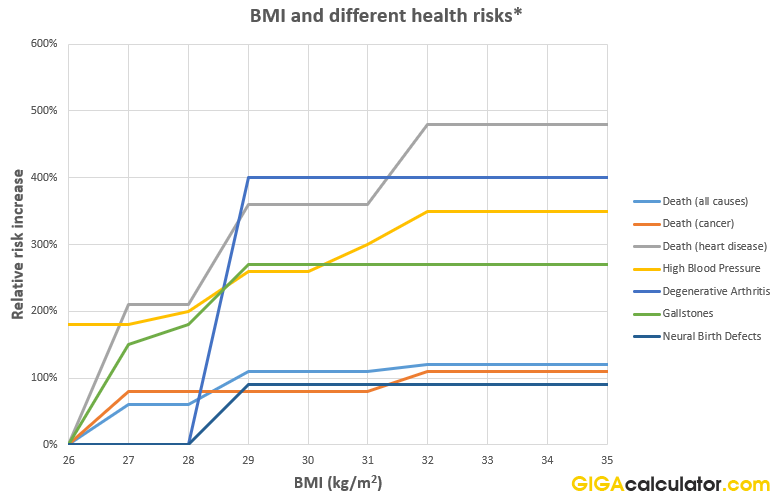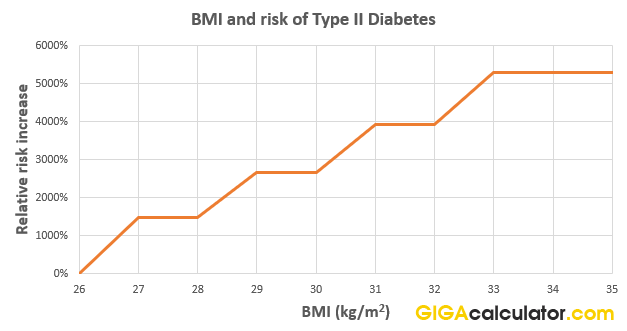# BMI Calculator

Use this online BMI calculator to easily calculate your Body Mass Index (BMI). It also shows your BMI category, as well as the BMI Prime index and how much overweight or underweight you are compared to the optimal weight range.

ft
in
Share calculator:

Embed this tool!
get code

## What is BMI?

Body Mass Index (BMI) is an index that attempt to quantify the amount of muscle, fat, and bone mass in an individual, and categorize them as underweight, normal weight, overweight, or obese, based on the index value. The simplicity of the body mass index calculation is one of its advantages: to calculate it one only needs to know his or hers weight and height. Our BMI calculator also requires just these two measurements as input and the output is in the units BMI is measured: kg per square meter (kg/m2), which is often left out in both writing and speech.

The concept of the BMI is due to one Adolphe Quetelet - a Belgian astronomer, mathematician, statistician and sociologist and his work on "social physics" between 1830 to 1850. The modern term "body mass index" (BMI) was coined in a paper published in the July 1972 edition of the Journal of Chronic Diseases by Ancel Keys et al.., in which it was argued that what was defined as BMI was "...if not fully satisfactory, at least as good as any other relative weight index as an indicator of relative obesity" .

The importance and usefulness of the index and hence any BMI calculator is in its curvilinear relationship to all-cause mortality. The bigger your calculated BMI is, the greater the risk for cardiovascular complications like hypertension, type II diabetes, some types of cancer, renal disease, degenerative arthritis, gallstones . See below for a list of BMI categories (classifications).

## BMI formula

The formula is simple:

BMI (kg/m2) = weight (kg) / height2 (m)

Of course, in our online BMI calculator you can enter either SI units or imperial metrics in feet in inches as well as pounds for weight - it does the conversions for you. Weight is referred to as "mass" in medical literature, while height is often called "stature".

## How to calculate your Body Mass Index?

So, how to calculate BMI knowling the formula above? It is straightforward task, especially if you already know your height and weight in meters and kg. Then just multiply your height by itself, and then divide your weight by the result. If you do not, some conversions might be necessary.

For example, if you are 5 feet 10 inches tall, that is 5 x 12 + 10 = 70 inches. 1 inch is 2.54 cm so you are 70 x 2.54 = 177.8 cm tall. 1 cm = 0.01 m, so you are 177.8 x 0.01 = 1.778 meters tall. 1.778 x 1.778 = 3.16

If you also weigh 170 pounds, that is 170 * 0.453 = 77 kg. 77 / 3.16 = 24.36, so your BMI is ~24, putting you just in the healthy range.

## BMI classes / categories

Individuals are classified in several BMI categories (classes) according to their mortality risk. There are different approaches to categorization, but below is the widely accepted WHO categorization. These are the classifications used in our BMI calculator as well.

Mean (average) BSA for males by age
CategoryBMI range (kg/m2)BMI PrimeRisk level
Very severely underweight less than 15 less than 0.60 Moderate
Severely underweight 15 - 16 0.60 - 0.64 Moderate
Underweight 16 - 18.5 0.64 - 0.74 Moderate
Normal (healthy) 18.5 - 25 0.74 - 1.0 Very low
Overweight 25 - 30 1.0 - 1.2 Low
Moderately obese 30 - 35 1.2 - 1.4 Moderate
Severely obese 35 - 40 1.4 - 1.6 High
Very severely obese 40+ 1.6+ Very high

As you can see, there is a moderate risk in being underweight, but certainly obesity is a primary risk factor for different sorts of causes for mortality. Using our BMI calculator, you will also get percentage over/under the normal limit as an output.

#### Graph - BMI health risks

The graph shows relationship between being overweight or obese according to BMI measures and relative risk of different diseases.* some health risks are calculated differently than others. Risk of death is versus BMI < 19, risk of type II diabetes is versus BMI < 22-23, high blood pressure vs BMI < 23, arthritis and gallstones are vs. BMI < 24, neural birth defects vs 19-27. This is because the data comes from different studies.

#### Graph - BMI and type II diabetes

Type II diabetes and its relationship to body mass index needed to be plotted separately due to the extremely high increases of relative risk per point - reaching 50 times increased relative risk at BMI 33-35.The data for both graphs is from reference 2 from the NCHS.

## What is BMI Prime?

BMI Prime is a mathematical transformation of the BMI index where you calculate the ratio between the actual BMI and the upper limit optimal BMI of 25. It is a dimensionless number and is unit-independent. Its main advantage is ease of use, e.g. if you are above 1.0 you know you are overweight and having a BMI Prime of 1.30 means you are 30% (0.3 * 100) above the optimum.

Since optimal BMI may vary between populations, the BMI Prime number is a useful comparison tool as it can be calculated differently for the different populations but result in the same scale. Our tool also serves as a BMI Prime calculator - just check the output.

## How reliable are BMI calculations?

BMI, like any other statistical measure applied to an individual, has its limitations and these apply to this BMI calculator as well. The especially noteworthy limitation concerns athletes in whom a high BMI can lead to incorrectly interpreting it as excess body fat. This happens with individuals with excessive muscle mass due to genetic makeup or training.

Large-size, field-event athletes, body-builders, weight lifters, heavy weight wrestlers and boxers, professional football players are all in risk of being misclassified. To take a U.S. example: the average BMI of the defensive linemen from a former NFL Super Bowl team averaged almost 32, placing these athletes in the "obese" category with moderate mortality risk - a clear illustration of the limitations in certain cases. The limitations apply to all BMI charts and modern body mass index online calculators.

#### References

 Katch V.L., McArdle W.D., Katch F.I (2011) "Essentials of Exercise Physiology", fourth edition

 US NCHS (2016) "National Health and Nutrition Examination Survey (2011-2014)" DHHS Publication No. 1604, s.3, N 39

 Keys A., Fidanza F., Karvonen M.J., Kimura N., Taylor H.L. (1972) "Indices of relative weight and obesity" Journal of Chronic Diseases 25 (6): 329–43

#### Cite this calculator & page

If you'd like to cite this online calculator resource and information as provided on the page, you can use the following citation:
Georgiev G.Z., "BMI Calculator", [online] Available at: https://www.gigacalculator.com/calculators/bmi-calculator.php URL [Accessed Date: 19 Jan, 2020].